Start typing, then use the up and down arrows to select an option from the list.# Dipole Moment Example 1

Jules Bruno
341views
3
2
we're going to say dipole moment is the polarity that arises when elements in a bond have a significant difference in their electoral negativity is not polarity itself. Is the unequal sharing of electrons between bonding Adams on We're gonna say a difference in electro negativity greater than 0.4 is considered significant now difference. Electro negativity abbreviated as Delta e n equals the larger or higher electro negativity value minus the smaller or lower electro negativity value, we're going to say the dipole. The type of moment is illustrated by dipole arrow that points towards the mawr electro negative value or element. So here we have carbon and we have flooring. If we look at this example, it says, calculate the difference in electro negativity. These values between carbon and flooring while carbon has an electoral negativity value of 2.5 and flooring is four point out. So Delta e n equals the larger value of 4.0, minus a smaller value of 2.5, so they're difference would be 1.5, which would give us option D. The fact that the electro negative negativity value is greater than 0. means that this bond is a polar bond and therefore would have a dipole moment. And a dipole moment means we have to use this die poll arrow. So that's how things are connected together. So if you're ever looking between two elements and you're trying to determine if the bond is polar and therefore has a dipole moment, you look at their difference and electro negativity one greater than 10.4 would mean that bond is polar and requires a dipole moment arrow. So again, because flooring is more electoral negative, the arrow would point towards it. Carbon is mawr is less electro negative mawr electoral positive. So if you think about it, this end here kind of looks like a positive sign if you were to circle it, and that's why it's on near carbon. So just keep this in mind when looking at the chemical bomb between two elements to determine if it has a dipole moment or not.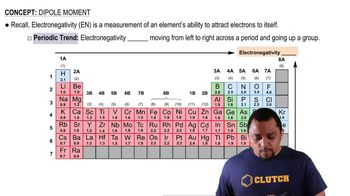01:33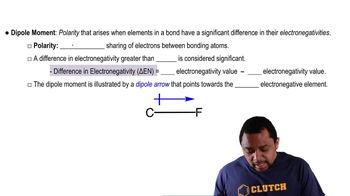01:25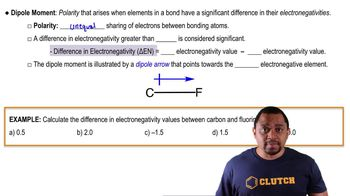02:14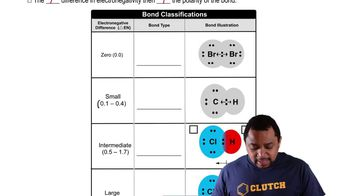03:18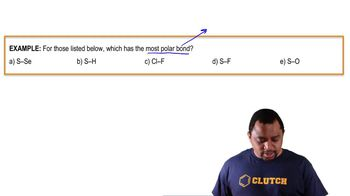01:07